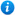# Year 4 Decimals Bundle

Year 4 Decimals Bundle

Year 4 maths programme of study - Number - fractions (including decimals)

• recognise and write decimal equivalents of any number of tenths or hundredths
• recognise and write decimal equivalents to 1/2, 1/4, 3/4
• find the effect of dividing a one- or two-digit number by 10 and 100, identifying the value of the digits in the answer as ones, tenths and hundredths
• round decimals with one decimal place to the nearest whole number
• compare numbers with the same number of decimal places up to two decimal places

This year 4 maths bundle introduces pupils to the curriculum objectives of the year 4 maths programme of study listed above. It contains 12 PowerPoint presentations with accompanying worksheets. These include:

• Tenths and Hundredths - Year 4
• Decimal Tenths - Year 4
• Place Value and Tenths - Year 4
• Decimal Tenths on a Number Line
• Dividing One and Two Digit Numbers by Ten - Year 4
• Decimal Hundredths - Year 4
• Place Value and Hundredths - Year 4
• Dividing One and Two Digit Numbers by 100 - Year 4
• Comparing Decimals - Year 4
• Ordering Decimals - Year 4
• Rounding Decimals - Year 4
• Halves, Quarters and Three Quarters as Decimals - Year 4

For a full description of each resource please click on the image of the included resources below.

Get access to thousands of pages of resources. Find out more about membership here.

Our Price : £9.99## Resources included (12)

£1.99
Tenths and Hundredths - Year 4
24 powerpoint slides | 3 worksheets
£1.99
Decimal Tenths - Year 4
23 powerpoint slides | 2 worksheets
£1.99
Place Value and Tenths - Year 4
37 powerpoint slides | 5 worksheets
£1.99
Decimal Tenths on a Number Line - Year 4
37 powerpoint slides | 4 worksheets
£1.99
Dividing One and Two Digit Numbers by Ten - Year 4
32 powerpoint slides | 4 worksheets
£1.99
Decimal Hundredths - Year 4
24 powerpoint slides | 3 worksheets
£1.99
Place Value and Hundredths - Year 4
37 powerpoint slides | 5 worksheets
£1.99
Dividing One and Two Digit Numbers by 100 - Year 4
32 powerpoint slides | 4 worksheets
£1.99
Comparing Decimals - Year 4
40 powerpoint slides | 5 worksheets
£1.99
Ordering Decimals - Year 4
38 powerpoint slides | 5 worksheets
£2.50
Rounding Decimals - Year 4
47 powerpoint slides | 5 worksheets

By continuing to use the site, you agree to the use of cookies. You can change this and find out more by following this link